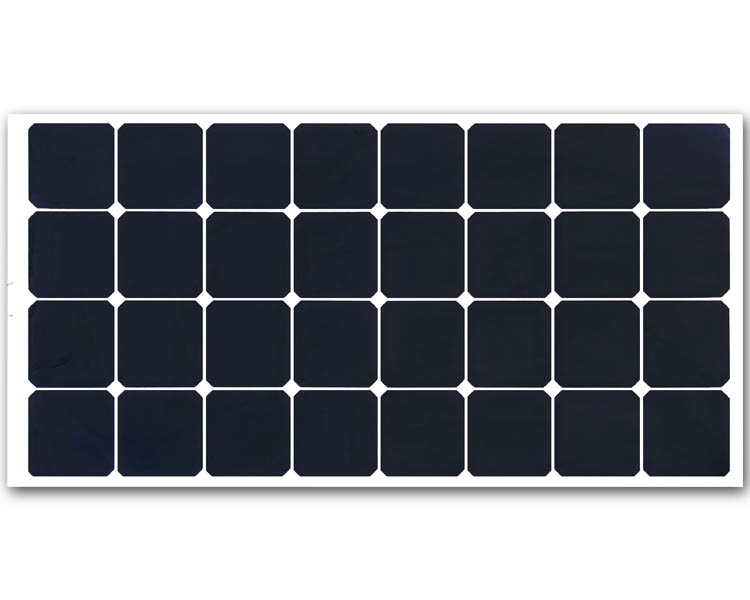News Details

# How much electricity can solar panel generate in a day?

Posted:2020/10/9 0:00:00 Tags： solar panel

Summary If you want to ask how much electricity a solar panel can generate in a day? Under the influence of many factors, it is actually difficult to give an absolute number.

If you want to ask how much electricity a solar panel can generate in a day? Under the influence of many factors, it is actually difficult to give an absolute number.

But we can make a theoretical calculation: a 100-watt solar panel can theoretically emit 100W*6H=600WH under standard lighting conditions (light intensity per square meter is 1000W) and an average sunshine duration of 6 hours. =0.6KWH=0.6 kWh of electricity.

After all, there is a gap between theory and reality. This figure must be discounted in real scenarios. If we calculate various losses (line loss, light angle, light intensity, etc.), we calculate with a conversion rate of 70%, A 100-watt solar panel can generate about 0.6*70%=0.42 kWh per day.